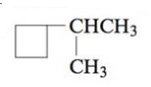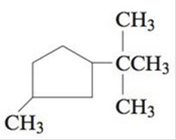Chapter 22, Problem 22E

Chapter
Section
Textbook Problem

# Name each of the following cyclic alkanes, and indicate the formula of the compound.a.b.c.(a)

Interpretation Introduction

Interpretation: The given compounds are to be named and their formula is to be indicated.

Concept introduction: Rules given by IUPAC should be followed to name an organic compound. Any organic compound has only one name that denotes that compound. The root word determines the number of carbons while counting the longest carbon chain. If more than one substituent are present, prefixes like di, tri, tetra, etc. are used and different substituents are written in alphabetical order.

Explanation

To determine: The names of the given organic compound and its chemical formula

The given compound is,

Figure 1

The ring is named as cyclobutane as it comprises of four carbon atoms...

(b)

Interpretation Introduction

Interpretation: The given compounds are to be named and their formula is to be indicated.

Concept introduction: Rules given by IUPAC should be followed to name an organic compound. Any organic compound has only one name that denotes that compound. The root word determines the number of carbons while counting the longest carbon chain. If more than one substituent are present, prefixes like di, tri, tetra, etc. are used and different substituents are written in alphabetical order.

(c)

Interpretation Introduction

Interpretation: The given compounds are to be named and their formula is to be indicated.

Concept introduction: Rules given by IUPAC should be followed to name an organic compound. Any organic compound has only one name that denotes that compound. The root word determines the number of carbons while counting the longest carbon chain. If more than one substituent are present, prefixes like di, tri, tetra, etc. are used and different substituents are written in alphabetical order.

### Still sussing out bartleby?

Check out a sample textbook solution.

See a sample solution

#### The Solution to Your Study Problems

Bartleby provides explanations to thousands of textbook problems written by our experts, many with advanced degrees!

Get Started